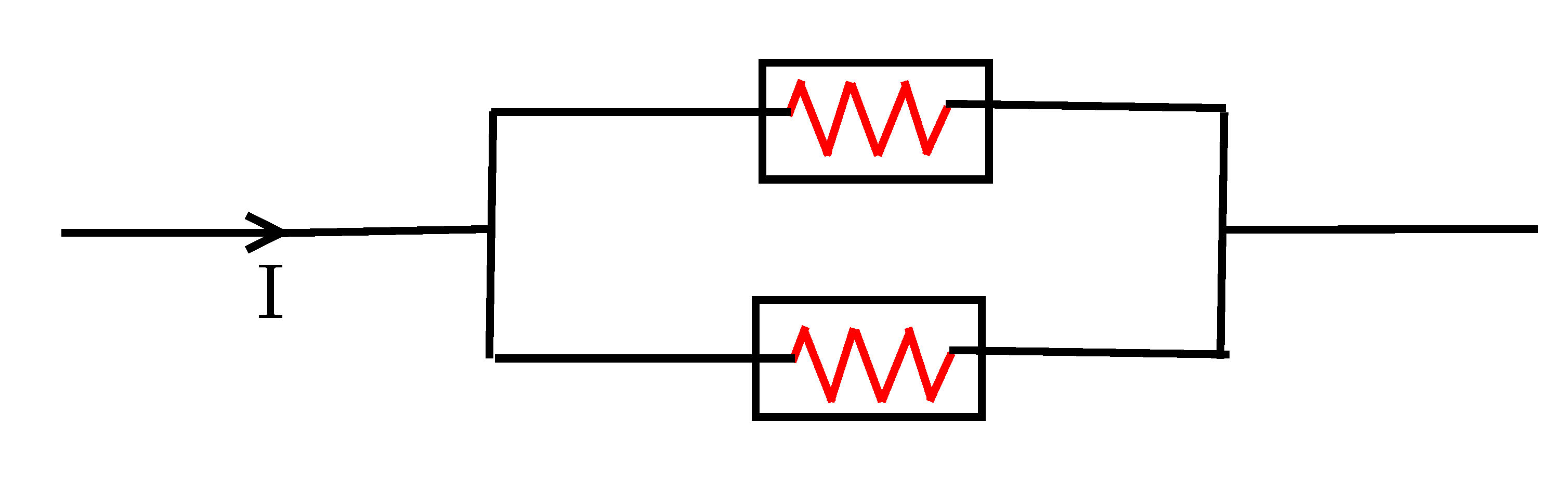# Connecting fuses in parallel

A fuse is a safety device used to protect an electrical circuit from the effects of excessive current. It consists of a thin metal wire with small resistance and low melting point. When an electrical equipment becomes faulty (allowing an excess electric current to flow through the circuit), the wire inside the fuse melts which breaks the flow of the current. The rated current $I_{N}$ is the maximum current that a fuse can conduct without interrupting the circuit. Consider two identical fuses with $I_{N}= 2~\mbox{A}$ connected in parallel as shown in the figure below.What is the maximum current $I$ in amps that can flow through the circuit?

×